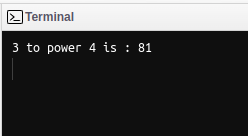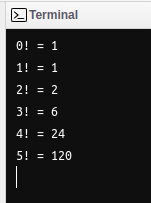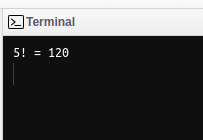GeeksforGeeks App
Open AppBrowser
Continue

## Related Articles

In the Lisp programming language, recursion is a commonly used technique for solving problems. Lisp is a functional programming language, which means it is well-suited to recursive solutions. In LISP, recursion is a programming technique in which a function calls itself repeatedly until a certain condition is met. It is a function that is called by itself more than once. This can be a useful way to solve large problems that can be broken down into smaller subproblems. A function is recursive if it calls itself –

• Boundary condition: then it’s not recursive
• Recursive condition: must be a smaller sub-problem to converge the solution.

In recursion, the recursive function ends until its base condition is satisfied. The base condition is a must in recursion otherwise the recursive function may go into an infinite loop which may cause it to memory’s stack overflow. A function that uses recursion is called a recursive function. The below figure shows the syntax and flow of the recursion in LISP.

### Syntax

(defun function_name(parameters);

body-of-function

if(base-condition)

return;

body-of-function

function_name(parameters)

)

Example 1:

## Lisp

 `(``defun` `power (x y)``  ``( ``if` `(``=` `y ``0``)              ``       ``1`                           `    ``(``*` `x (power x(``-` `y ``1``)))``   ``)`` ``) ``   ` `(``format` `t` `"3 to power 4 is : ~D"` `(power ``3` `4``))`

Output :The above recursive power function computes 3 to power 4 i.e (3 * 3 * 3 * 3) = 81.

The Factorial of a number n is the product of all the numbers from 1 to 5. Here is a recursive implementation of a function that calculates the factorial of a given number.

Example 2:

## Lisp

 `(``defun` `factorial (n)``  ``(``if` `(``=` `n ``0``)``      ``1``      ``(``*` `n (factorial (``-` `n ``1``))) ) )`` ` `(``loop` `for i from ``0` `to n``   ``do (``format` `t` `"~A! = ~A~%"` `i (factorial i)) )`

Output:This function works by first checking if the input ‘n’ is zero. If it is, the function returns 1. If ‘n’ is not zero, the function calls itself with an input of (n-1), which is one less than n. This recursive call continues until ‘n’ is reduced to zero, at which point the recursive calls will start returning and the final result will be calculated. To calculate the factorial of 5, the function would be called with an input of 5 and would perform the following calculations and the flow of the recursive function would be:

```(* 5 (factorial 4))
(* 5 (* 4 (factorial 3)))
(* 5 (* 4 (* 3 (factorial 2))))
(* 5 (* 4 (* 3 (* 2 (factorial 1)))))
(* 5 (* 4 (* 3 (* 2 (* 1 (factorial 0))))))
(* 5 (* 4 (* 3 (* 2 (* 1 1)))))
(* 5 (* 4 (* 3 (* 2 1))))
(* 5 (* 4 (* 3 2)))
(* 5 (* 4 6))
(* 5 24)
---> 120```

## LISP Tail Recursion

LISP also has a built-in function called ‘recur’ that can be used to implement Tail-recursive functions. A tail-recursive function is a special type of recursive function in which the recursive call is the last thing that the function does before returning a result. This allows the interpreter to optimize the function and avoid building up a large call stack, which can improve performance and reduce the risk of running out of stack space.

Example 3:

## Lisp

 `(``defun` `factorial (n acc)``  ``(``if` `(``=` `n ``1``)``      ``acc``      ``(recur (``-` `n ``1``) (``*` `acc n))))`` ` `(``format` `t` `"Factorial of 5 is ~D"` `(factorial ``5` `1``))`

Output:This function works in the same way as the previous example, but it uses an accumulator variable ‘acc’ to keep track of the intermediate results. The initial value of ‘acc’ should be ‘1’.

There are many different types of problems that can be solved using recursion and traditional method using functions. A recursion is a powerful tool for solving problems in a concise way.  However, it is important to use recursion carefully, as it can lead to infinite loops if not implemented correctly. Overall, recursion is a valuable technique for a programmer.

My Personal Notes arrow_drop_up